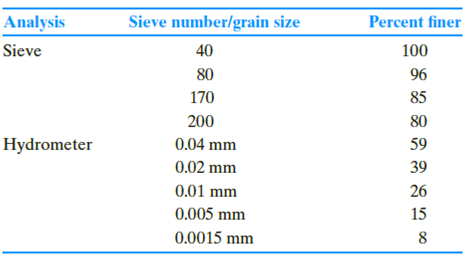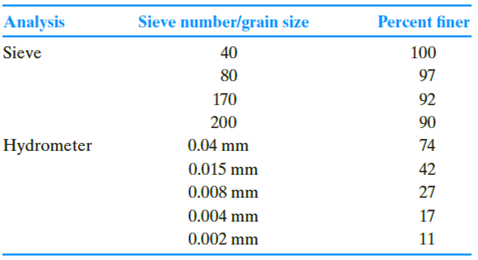Chapter 2, Problem 2.9PPrinciples of Geotechnical Enginee...

9th Edition
Braja M. Das + 1 other
ISBN: 9781305970939

Solutions

Chapter
SectionPrinciples of Geotechnical Enginee...

9th Edition
Braja M. Das + 1 other
ISBN: 9781305970939
Textbook Problem

Repeat Problem 2.8 using the following data.2.8 The following are the results of a sieve and hydrometer analysis.a. Draw the grain-size distribution curve.b. Determine the percentages of gravel, sand, silt and clay according to the MIT system.c. Repeat Part b according to the USDA system.d. Repeat Part b according to the AASHTO system.

(a)

To determine

Draw the grain-size distribution curve.

Explanation

Given Information:

The sieve and hydrometer analysis are given in the Table.

The percent finer value for corresponding grain size is given in Table.

Calculation:

Plot the grain size distribution curve using grain size versus percent finer...

(b)

To determine

Calculate the percentages of gravel, sand, silt, and clay according to the MIT system.

(c)

To determine

Calculate the percentages of gravel, sand, silt, and clay according to the USDA system.

(d)

To determine

Calculate the percentages of gravel, sand, silt, and clay according to the AASTHO system.

Still sussing out bartleby?

Check out a sample textbook solution.

See a sample solution

The Solution to Your Study Problems

Bartleby provides explanations to thousands of textbook problems written by our experts, many with advanced degrees!

Get Started

What are the basic characteristics of nonlinear models?

Engineering Fundamentals: An Introduction to Engineering (MindTap Course List)

Should Gladys call the legal authorities? Which agency should she call?

Principles of Information Security (MindTap Course List)

What specific information do you need to create a work breakdown structure?

Systems Analysis and Design (Shelly Cashman Series) (MindTap Course List)

Explain issues surrounding colleges teaching students how to hack.

Enhanced Discovering Computers 2017 (Shelly Cashman Series) (MindTap Course List)